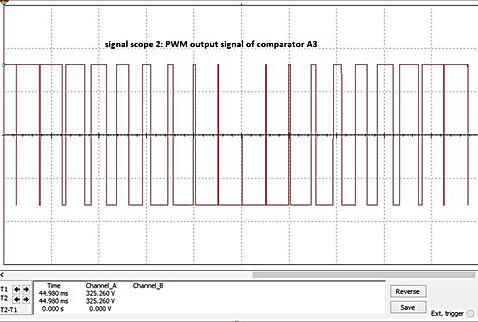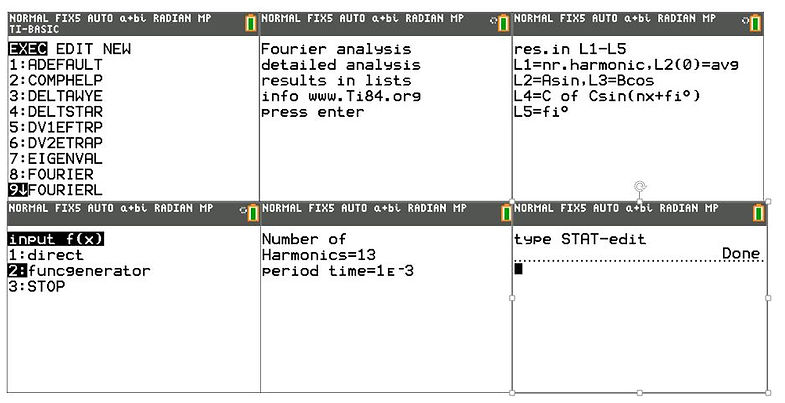naver-site-verification: naver47238a1b6bfbb19a2fd4b619734fa9a6.html
top of page

# PWM ( Puls Width Modulation) with the Ti-84 plus

## Generating  a PWM signal with the Ti84 plus CE

Generating a PWM signal in practice, a low-frequency sinusoidal signal is compared with a high-frequency triangle signal. The two signals are connected to a comparator. In our example, both the amplitudes of sinusoidal and triangle signal have a peak voltage of 1 V. The sinusoidal voltage has a frequency of 50 Hz and the triangle voltage has a frequency of 1 kHz.

In our Multisim circuit below, we use 2 comparators to achieve a high gain. The output signal of the comparator is high and positive if the sinusoidal signal is higher than the triangle signal, and high and negative if the sinusoidal signal is lower than the triangle. See the circuit and output of Scope 1 and Scope 2 below. The maximum output Voltage of the second comparator is set to 325.26V and -315.26V, which is the peak voltage of a 230V RMS  sinusoidal signal.## How to generate a PWM-signal with the Ti-84 calculator?

We demonstrated that, in practice, a PWM signal can be constructed in a relatively straightforward manner. Now we wish to generate a PWM signal using the Ti-84 plus calculator. Defining a sinusoidal signal with the Ti-84 is very simple, but a triangle signal can not be generated directly.

With the function generator program FUNCGEN.8xp, available on this website, a triangle function can be defined for a maximum of two to three periods. If the low-frequency sinusoidal voltage has a frequency of 50 Hz and the triangle signal has a frequency of 1 kHz, then at least 20 periods of the triangle signal for 1 period of the 50Hz sinusoidal signal are needed.

Using the PWM signal as the input signal for the differential equation solvers, at least two or three periods of the PWM signal are needed.

To approach the triangle, a function with terms of the Fourier series of the triangle function has been developed in order to generate a high-frequency triangle signal.

We will first investigate how many terms are need to achieve a good approximation of the triangle function. Using the Function generator program of this website, a triangle signal (1 period) is generated and analyzed using the Fourier program to calculate the Fourier series of the triangle signal.

## Fourier series triangle function

To calculate the Fourier series, we first define a triangle function with a period time of 1 msec (1kHz) and an amplitude of 1 using the program FUNCGEN.8xp. See the results belowThis triangle signal is analyzed with the Fourier program to determine the harmonicsThe results show that only odd sinusoidal functions (L2)are present, so the triangle can be approached by: 0.7994sin(2pi1000x)-.094sin2pi3000x)+.0324sin(2pi5000x)-,017sin(2pi7000x)+0.01sin(2pi9000x)-.007sin(2pi11000x) which looks to be a sufficient number of terms. The error between the real triangle and the signal made with the Fourier series has been investigated.

Below the comparison between real triangle and the Fourier series up to 11 harmonics. The error in amplitude is about 5 %. Which is acceptable for using it for the realizing of the PWM signal.## Generating the PWM signal with the Ti-84

Now a good approximation of the triangle function is available and the PWM signal is generated as follows, first the definition is shown in the Y-editor, then for 2 msec (zoom) the triangle is compared with the sinusoidal signal.

At the end, the PWM signal with a base frequency of 50 Hz) is shown.A special program has been written in Ti-Basic language (PWM.8xp) to generate a PWM signal with a desired voltage level and desired modulation index m =Vtopsinus/Vtoptriangle. In the example above, the peak value of the sinusoidal function of 1V and a top of the triangle function approached by Fourier of 0.95V. So, we have a modulation index of 1/0.95= 1.05.  The PWM signal generated with the program can be used as input in the differential equation solvers and in the Fourier programs.

## The program PWM.8xp

Based on the theory above, a program which generates a PWM signal has been written.The desired PWM signal is saved in Y6 just as the signals made with the Function generator program and can therefore be used as input signal for several programs such as first order differential equation solver DV1FTRP3.8xp.

## A PWM voltage supply loaded with a RL circuit

It is known that a PWM signal contains harmonic signals with a base frequency + multiple frequencies around the switching frequency of the triangle signal. So if the base frequency equals 50 Hz and the triangle frequency equals 1000 Hz, then the Fourier series contains signals of 50 Hz and around N*1000Hz. Connecting this PWM voltage on a RL circuit results in a current with contains mainly the base frequency. We choose for R to be 5 Ohm and L to be=0.5H. The inductance has an impedance of 157.1j Ohm for 50 Hz and 3141.6j Ohm for 1000Hz, so the inductance blocks the higher frequencies of the PWM voltage. See the figures below simulated with Multisim. Now, we are going to simulate this problem using the Ti-84## Simulation of the PWM Voltage Supply with an RL-circuit using the Ti-84

For the simulation, we use the program DV1FTRP3.8xp with the PWM signal generated with PWM.8xp as input. The results show that he Ti results agree very well with the Simulink Simulations.For a Free version of the program DV1EFTRP.8xp, send a mail to